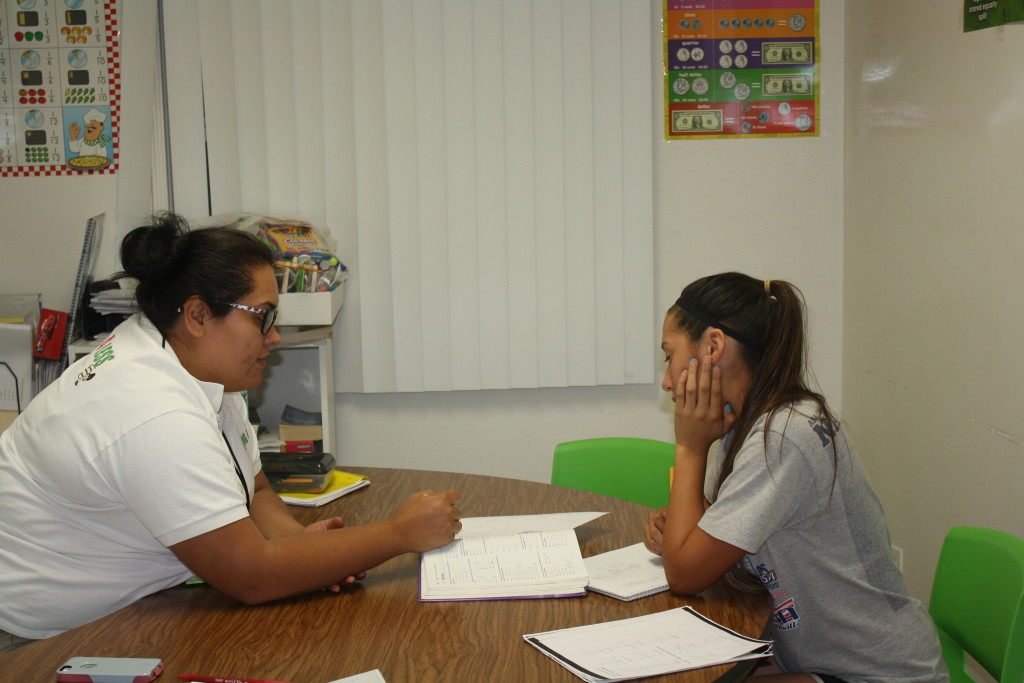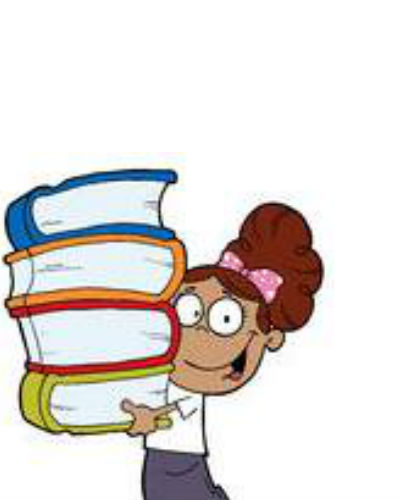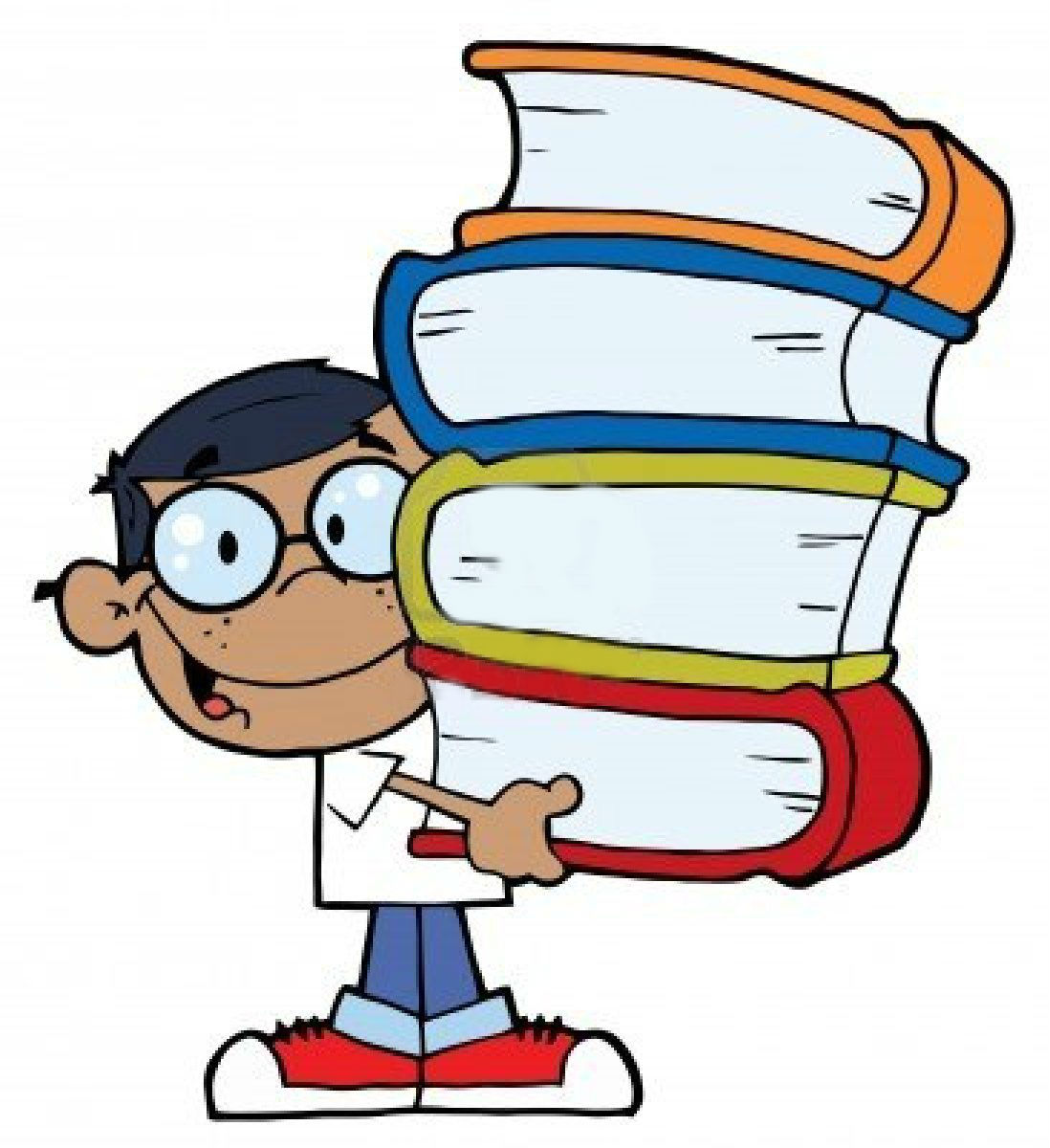#Algebra 2 is a course in mathematics that is taken by approximately 85% of all graduating high school seniors. The Algebra 2 Program at Tutoring 4 Less is designed to develop a student’s basic and advanced algebraic tools and their mathematical ability. Our program will also enable students to expertly participate in our ever more technology-driven society. Our tutors engage students in small group or one-on-one settings to deepen their understanding of Algebra 2 and the concepts it requires.

Students in the Algebra 2 program develop a firm grasp of the underlying mathematical concepts while using algebra, geometry, and trigonometry as tools for solving more complex problems.

## Best Algebra 2 Tutors Near Me in Los Angeles

When you begin to look for math tutors in Los Angeles, CA, you can run into stumbling blocks. Some tutors lack tutoring experience with your student’s learning style, and use the same lesson plans for tutoring students regardless of the student’s ability or preferences. Tutoring 4 Less circumvents these problems by choosing to work with quality tutors that have years of experience in teaching and tutoring elementary, middle school, and high school students.

## Online Tutoring For Algebra 2

Tutoring 4 Less offers has developed robust online tutoring options for Algebra 2. If your student is not getting the support they need in their high school math classes, our online Algebra 2 tutors can provide support, go through practice problems, or even revise previous concepts in order to better prepare your student for the rigors of Algebra 2. While many students are struggling with remote learning, others are using this opportunity to advance their math skills by taking advantage of online tutoring. Online tutoring is available to students in Los Angeles county, as well as nationwide and even worldwide! Algebra 2 doesn’t have to be a class your child struggles with, if they get the right support with online tutoring.## What Are the Topics in Algebra 2?

Algebra 2 focuses on the mastery of the analysis and graphing of polynomials, logarithmic and exponential functions, trigonometric identities, functions, and their applications, imaginary and complex numbers, conic sections, and matrices.

The previous levels of our mathematics program (Pre-algebra, Geometry, Algebra) help students build a solid foundation of linear equations, inequalities, and functions. Algebra 2 continues to build on this foundation by furthering the students knowledge of learned material and using Algebra 2 concepts to begin answering complex questions.

Below is a more detailed view of the topics covered in Algebra 2:

• Systems of Equations and Inequalities: In real-world applications (word problems) the systems of equations and inequalities that students learn about are important to help solve problems by using different methods such as graphing, elimination/addition, and substitution. Students are taught to write systems of equations on their own by deciphering word problems and breaking down the mathematical language into equations. In order to solve the systems students use one of the many methods available to determine the values or range of values (inequalities) that will make the set of equations true
• Functions and their Graphs: In this section of Algebra 2 students are taught how relationships and associations between numbers, sets of numbers, and functions are applied to graphing and interpreting graphs and their functions.
• Polynomial and Rational Functions: In this section students are taught how to factor, simplify expressions, and how to use the quadratic formula to find solutions. They are also introduced to higher power equations and the use of the Order of Operations along with the rules of factoring quadratics and binomials to solve complex and multi-step problems.
• Exponential and Logarithmic Functions: This section is an expansion on exponents. In the simplest form, logarithms are the inverse operators to exponents. Taking a log of a function tells the student what exponent one needs for the given base to get the given answer. Students are also introduced to interest problems, and exponential growth and decay (population growth, half-lives, & radioactive materials).
• Complex and Imaginary Numbers: This is a new concept that students are taught in Algebra 2. Here they learn about imaginary numbers, i, and how to factor, graph, and rationalize these imaginary values.
• Conics: Conics is an extension of graphing. Here students learn that conics are formed when planes intersect with cones, resulting in circles, ellipses, and hyperbolas. Students are also taught how to solve for the foci, vertex, and asymptotes.
• Matrices: Matrices are ways of organizing numbers. They are used extensively in computer simulations, graphics, and information processing.